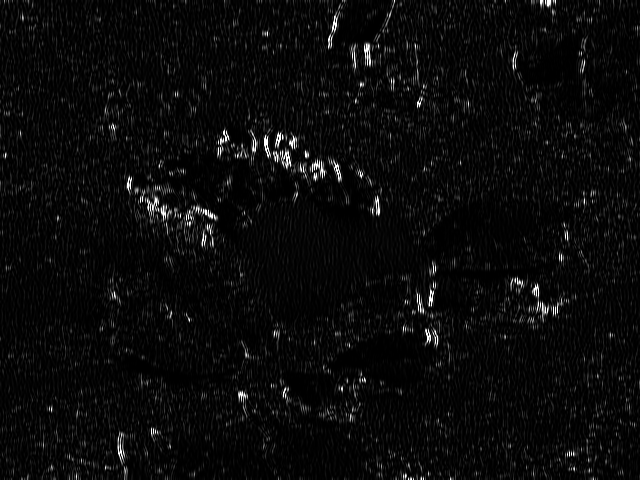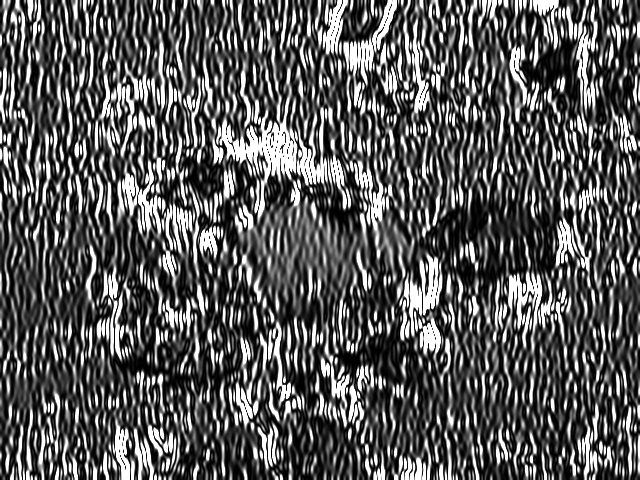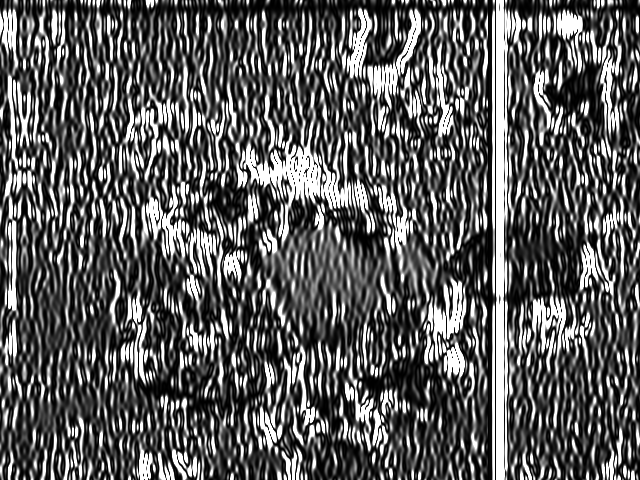# Revision history [back]

### gpu::convolve and gpu::filter2D vs cv::filter2D, opencv 2.4.9

I'm trying to use the opencv gpu module to filter an image with Gabor kernels. To check if everything is correct, I'm comparing the result of the CUDA accelerated filtering, with the regular CPU filtering. The code I'm using is available here: https://github.com/juancamilog/gpu_convolve_test.git

Since the funtcion cv::gpu::filter2D is limited to kernels of size smaller than 16x16, I'm using cv::gpu::convolve for larger kernels. In that case, I use cv::gpu::copyMakeBorder to produce a filter response that has the same size as the original image.

The problem I'm facing is that the result of the cv::gpu::filter2D is different from the result of cv::filter2D. This is even more noticeable when using the cv::gpu::convolve function. What is the cause of this difference? How do we obtain a GPU filtering response that is the same as the CPU filtering response?

### gpu::convolve and gpu::filter2D vs cv::filter2D, opencv 2.4.9

I'm trying to use the opencv gpu module to filter an image with Gabor kernels. To check if everything is correct, I'm comparing the result of the CUDA accelerated filtering, with the regular CPU filtering. The code I'm using is available here: https://github.com/juancamilog/gpu_convolve_test.git

Since the funtcion cv::gpu::filter2D is limited to kernels of size smaller than 16x16, I'm using cv::gpu::convolve for larger kernels. In that case, I use cv::gpu::copyMakeBorder to produce a filter response that has the same size as the original image.

The problem I'm facing is that the result of the cv::gpu::filter2D is different from the result of cv::filter2D. This is even more noticeable when using the cv::gpu::convolve function. What is the cause of this difference? How do we obtain a GPU filtering response that is the same as the CPU filtering response?

UPDATE Some example results for the cv::gpu::filter2D case (the differences are very subtle):And for the cv::gpu::convolve case:### gpu::convolve and gpu::filter2D vs cv::filter2D, opencv 2.4.9

I'm trying to use the opencv gpu module to filter an image with Gabor kernels. To check if everything is correct, I'm comparing the result of the CUDA accelerated filtering, with the regular CPU filtering. The code I'm using is available here: https://github.com/juancamilog/gpu_convolve_test.git

Since the funtcion cv::gpu::filter2D is limited to kernels of size smaller than 16x16, I'm using cv::gpu::convolve for larger kernels. In that case, I use cv::gpu::copyMakeBorder to produce a filter response that has the same size as the original image.

The problem I'm facing is that the result of the cv::gpu::filter2D is different from the result of cv::filter2D. This is even more noticeable when using the cv::gpu::convolve function. What is the cause of this difference? How do we obtain a GPU filtering response that is the same as the CPU filtering response?

UPDATE Some example results for the cv::gpu::filter2D case (the differences are very subtle):

CPUGPUAnd for the cv::gpu::convolve case:

CPUGPU### gpu::convolve and gpu::filter2D vs cv::filter2D, opencv 2.4.9

I'm trying to use the opencv gpu module to filter an image with Gabor kernels. To check if everything is correct, I'm comparing the result of the CUDA accelerated filtering, with the regular CPU filtering. The code I'm using is available here: https://github.com/juancamilog/gpu_convolve_test.git

Since the funtcion cv::gpu::filter2D is limited to kernels of size smaller than 16x16, I'm using cv::gpu::convolve for larger kernels. In that case, I use cv::gpu::copyMakeBorder to produce a filter response that has the same size as the original image.

The problem I'm facing is that the result of the cv::gpu::filter2D cv::gpu::convolve function is different from the result of cv::filter2D. This is even more noticeable when using the cv::gpu::convolve function. What is the cause of this difference? How do we obtain a GPU filtering response that is the same as the CPU filtering response?

UPDATE ( iwas hallucinating the differences in the results of filter2d Some example results for the cv::gpu::filter2D case (the differences are very subtle):(no difference):

CPUGPUAnd for the cv::gpu::convolve case:

CPUGPU### gpu::convolve and gpu::filter2D vs cv::filter2D, opencv 2.4.9

I'm trying to use the opencv gpu module to filter an image with Gabor kernels. To check if everything is correct, I'm comparing the result of the CUDA accelerated filtering, with the regular CPU filtering. The code I'm using is available here: https://github.com/juancamilog/gpu_convolve_test.git

Since the funtcion cv::gpu::filter2D is limited to kernels of size smaller than 16x16, I'm using cv::gpu::convolve for larger kernels. In that case, I use cv::gpu::copyMakeBorder to produce a filter response that has the same size as the original image.

The problem I'm facing is that the result of the cv::gpu::convolve function is different from the result of cv::filter2D. What is the cause of this difference? How do we obtain a GPU filtering response that is the same as the CPU filtering response?

UPDATE ( iwas hallucinating the differences in the results of filter2d Some example results for the cv::gpu::filter2D case (no difference):

CPUGPUAnd for the cv::gpu::convolve case:

CPUGPU